# Frank Solutions Class 9 Maths Chapter 20 Constructions of Quadrilaterals

Frank Solutions Class 9 Maths Chapter 20 Constructions of Quadrilaterals describe the important steps to be followed in the construction of quadrilaterals. The solutions are explained accurately to boost exam preparation among students. They can clear their doubts by referring to Frank Solutions. The Frank Solutions Class 9 Maths Chapter 20 Construction of Quadrilaterals PDF can be downloaded for free from the link available below.

Chapter 20 explains the steps of construction in depth from the exam perspective, as prescribed by the ICSE board. Following these solutions while solving textbook problems helps students to learn the concepts effectively. They can also analyse their areas of weaknesses, which need to be focused more upon during exam preparation.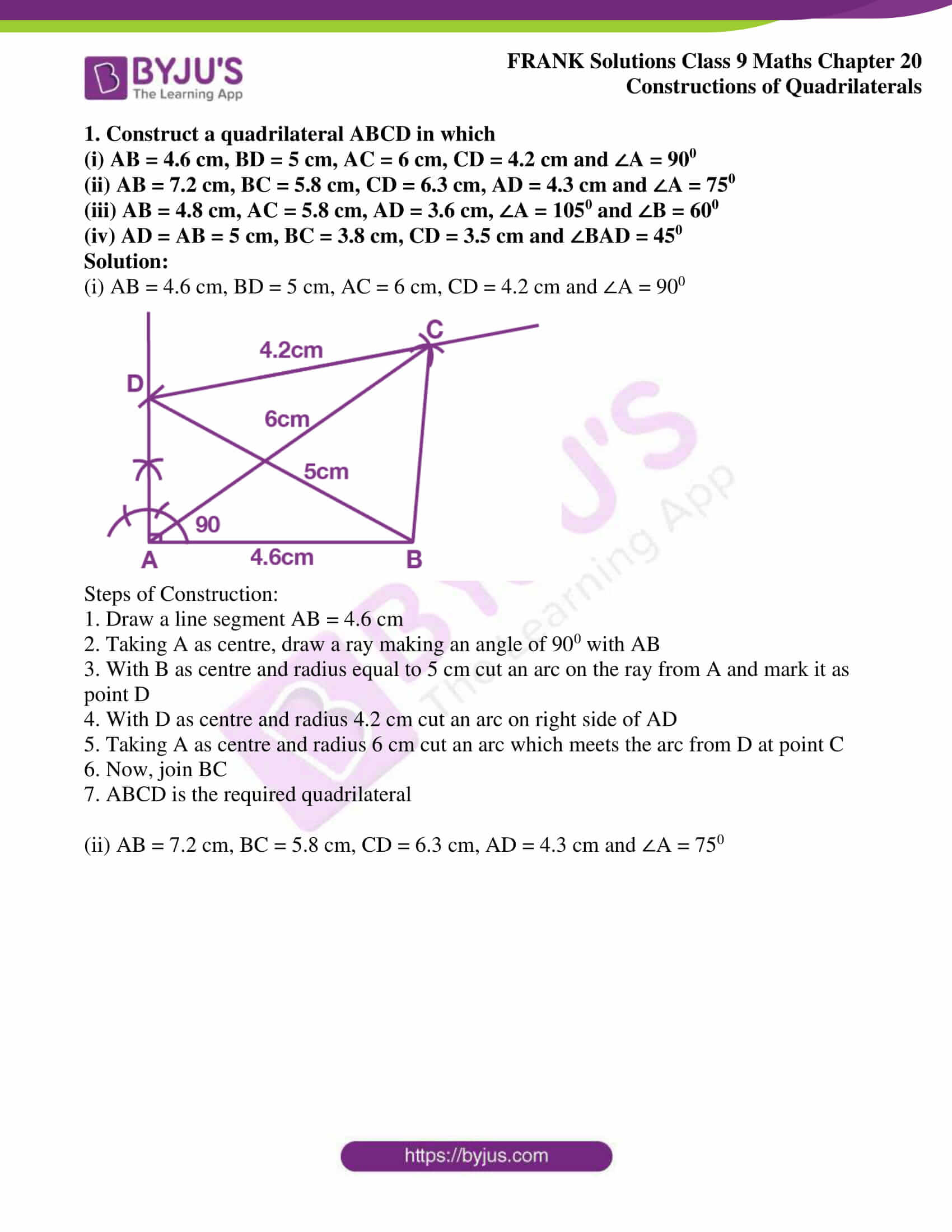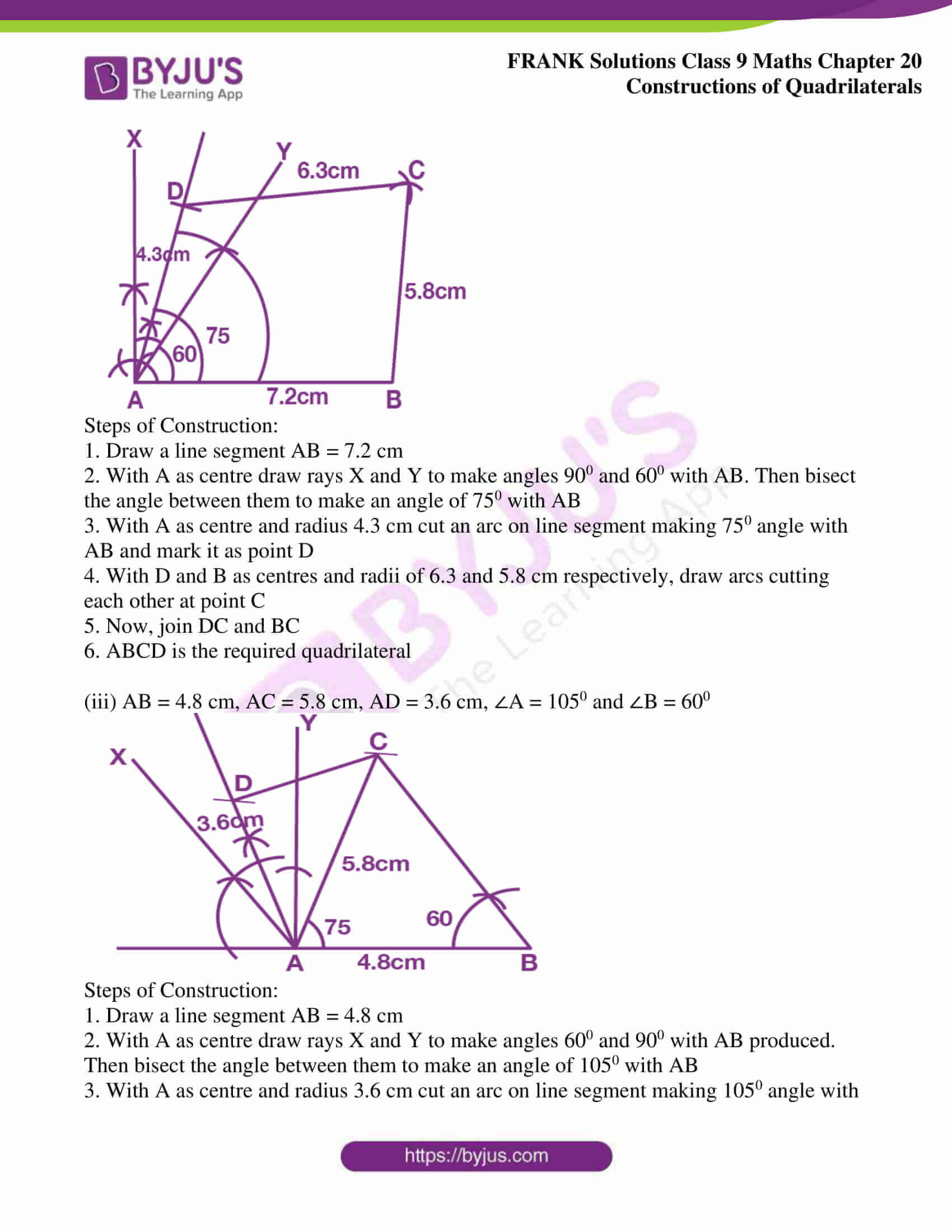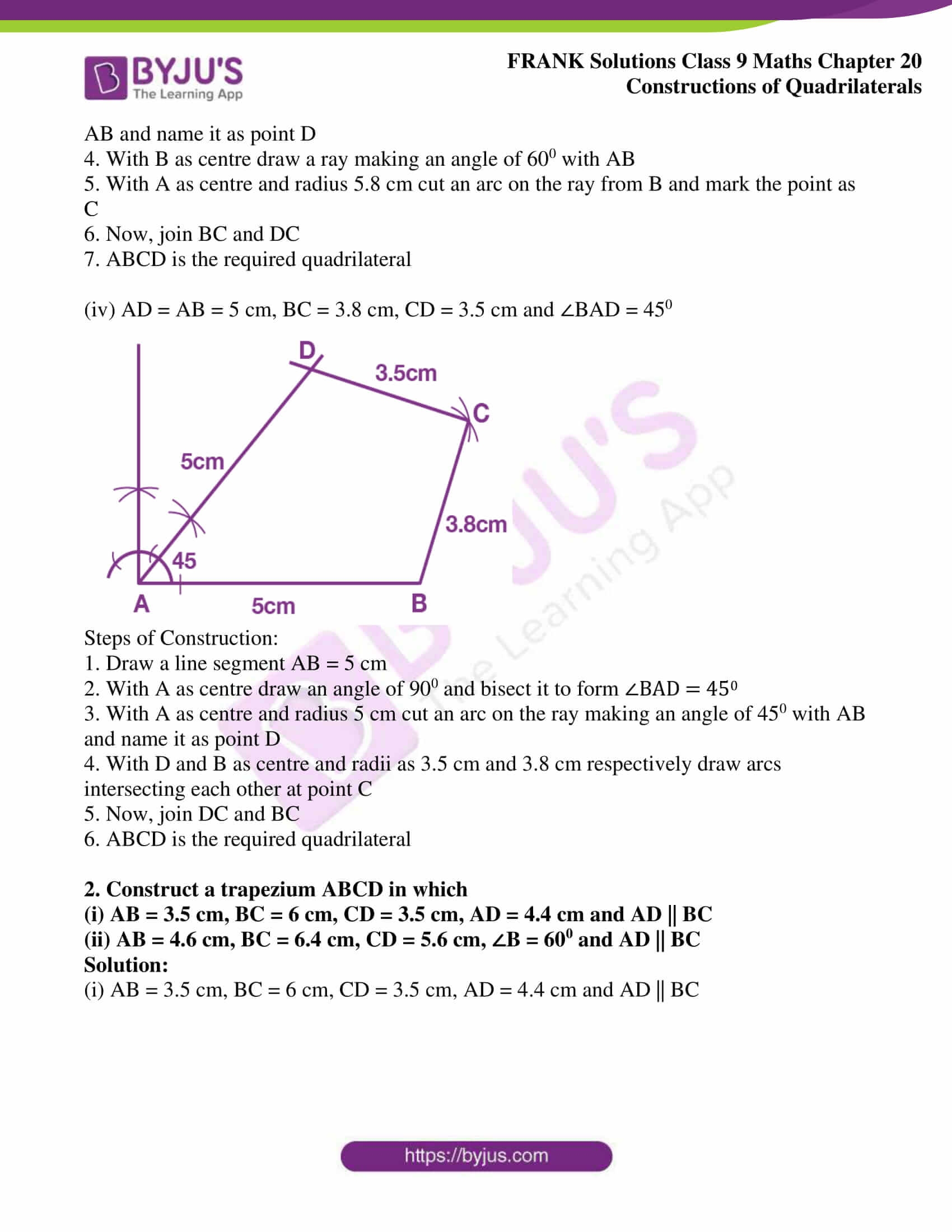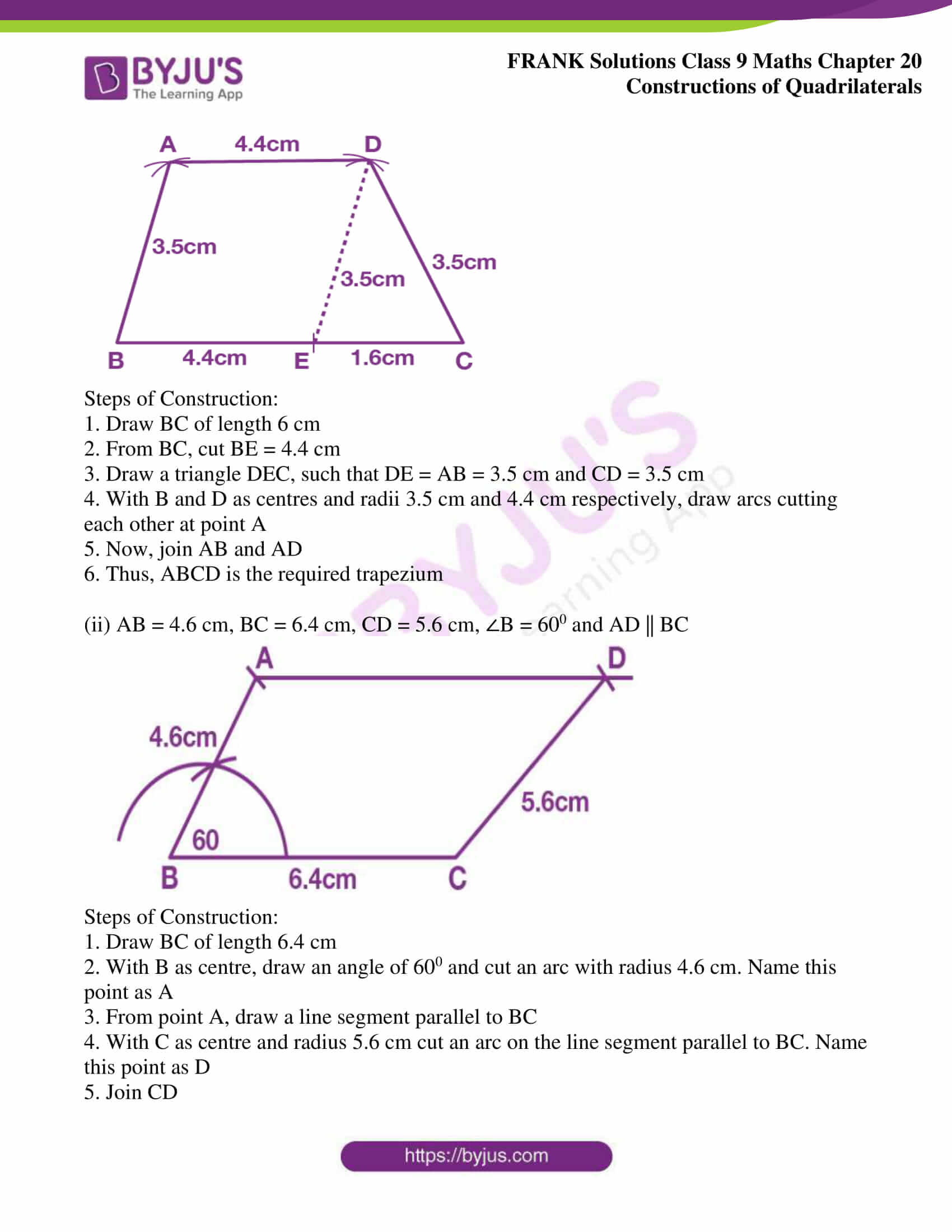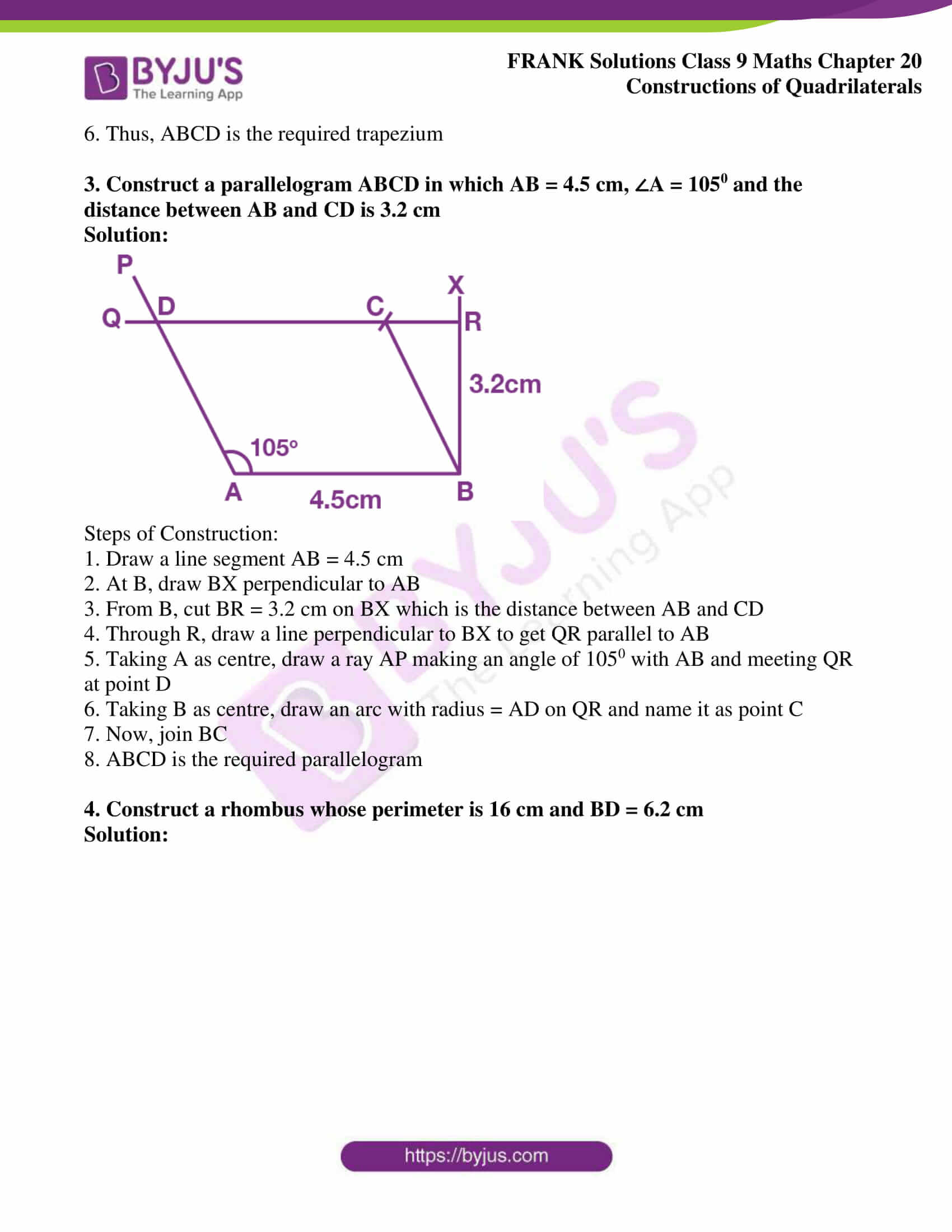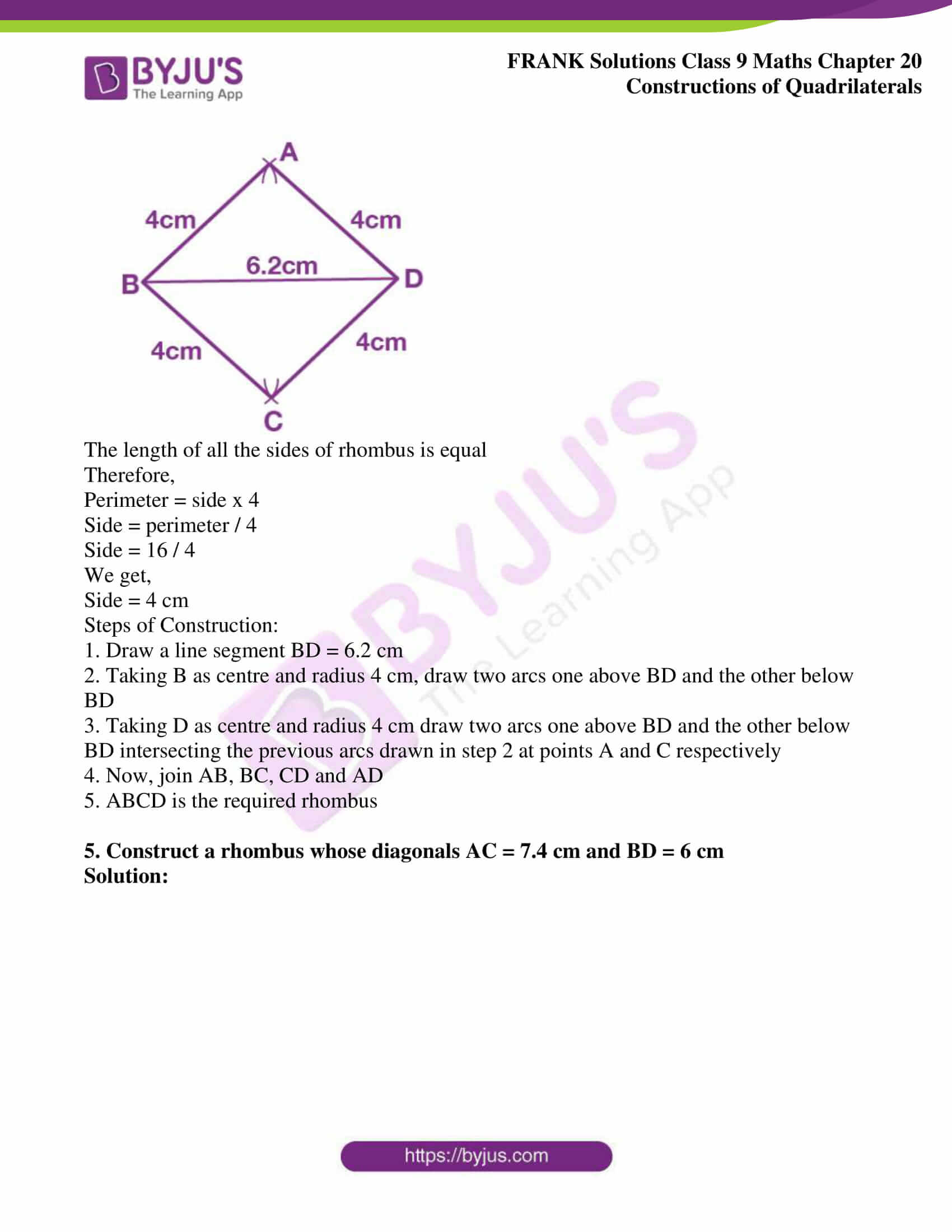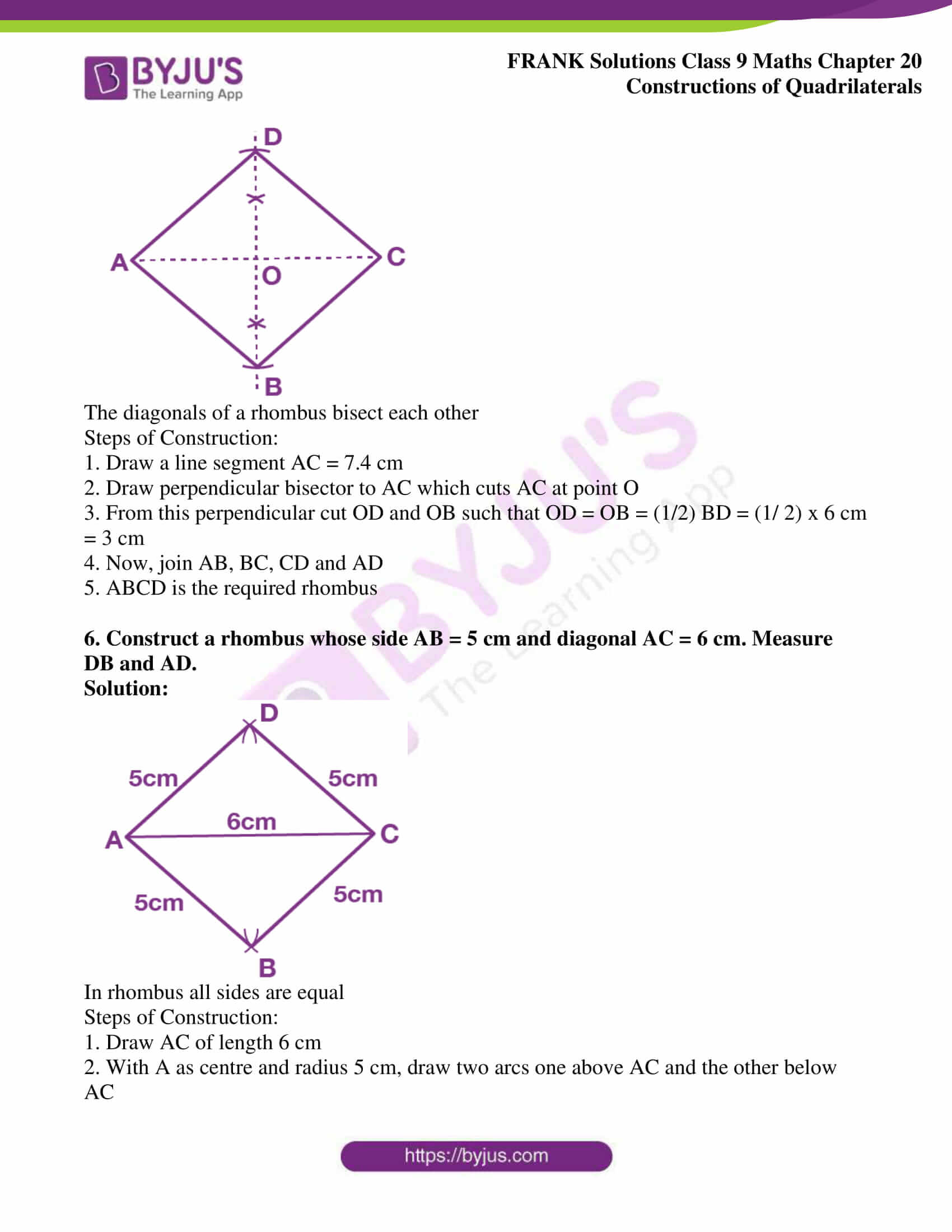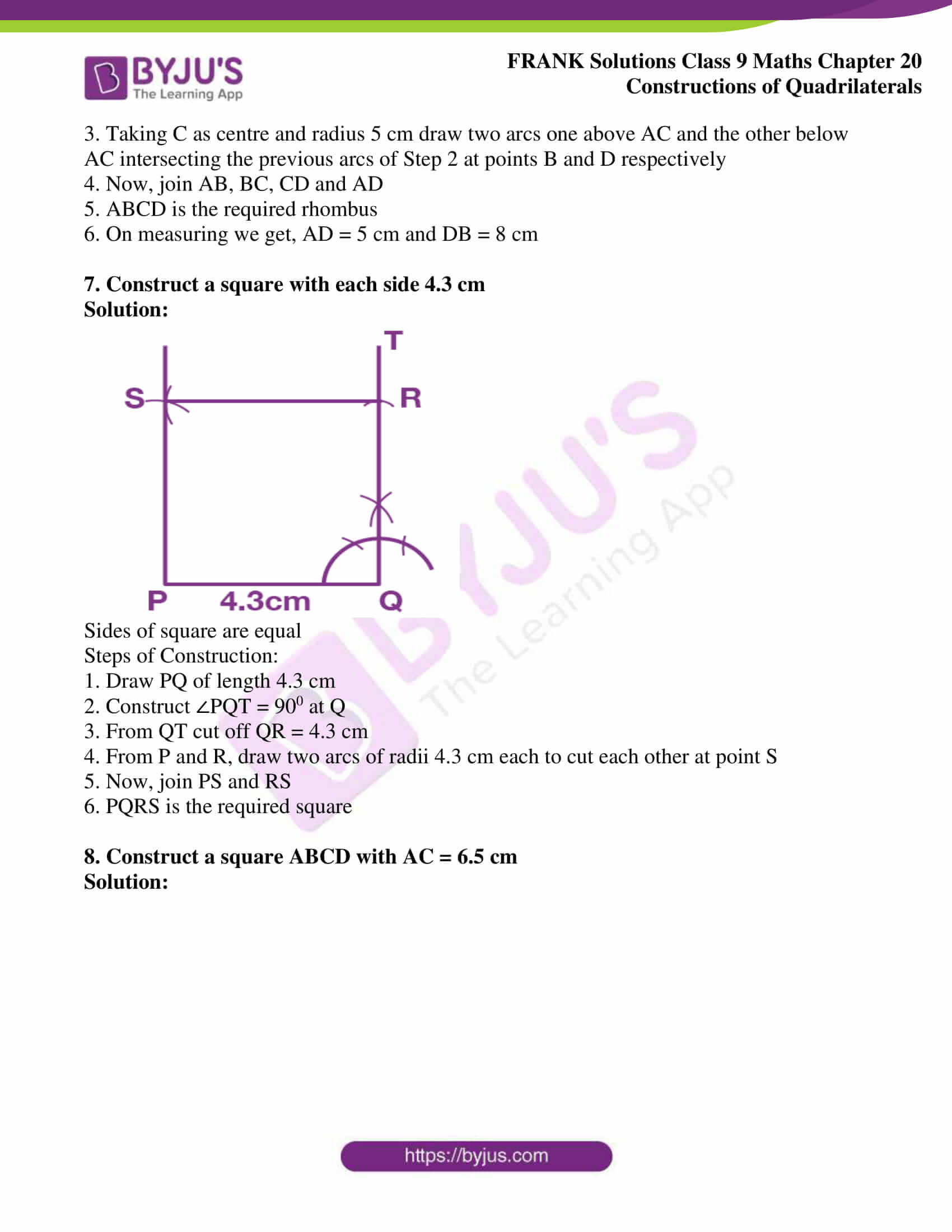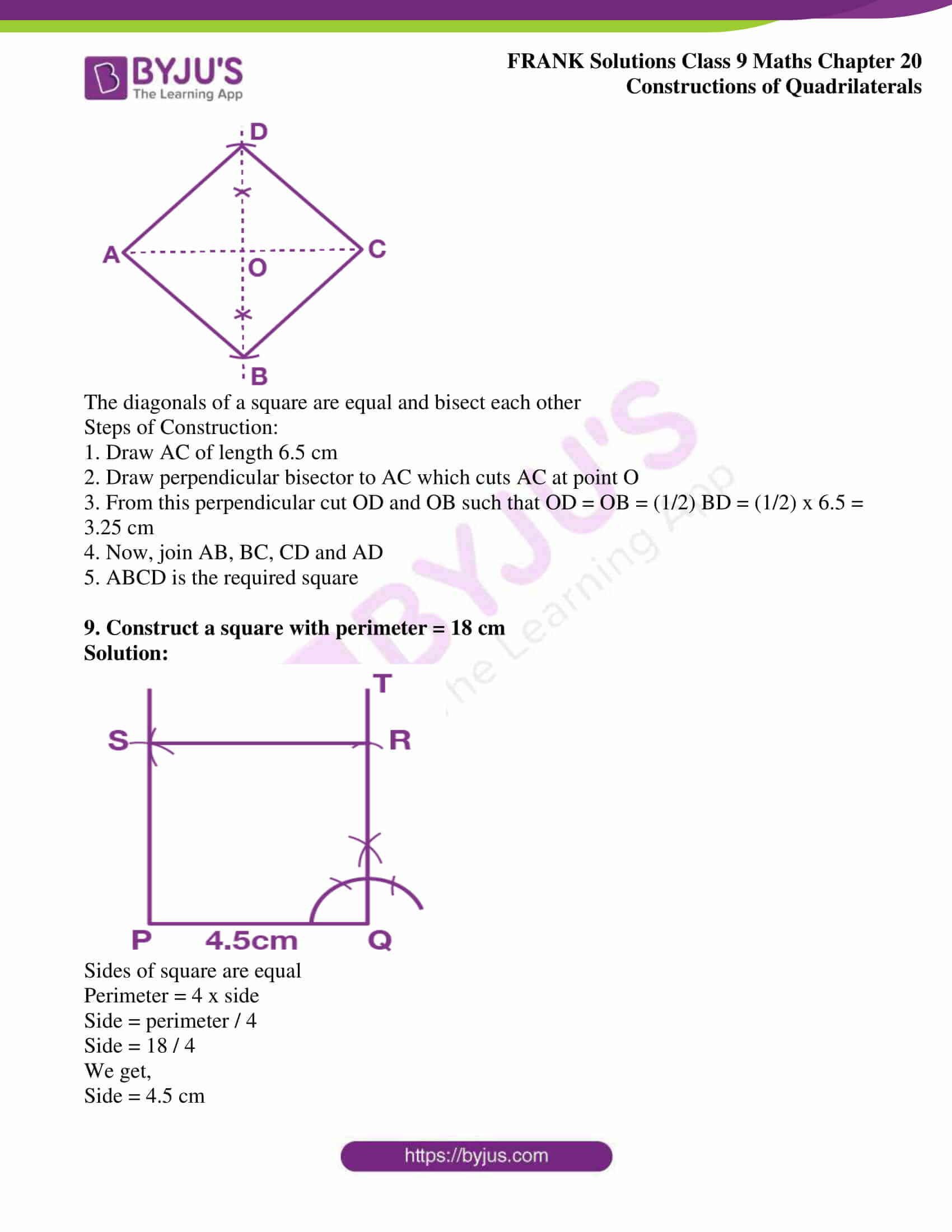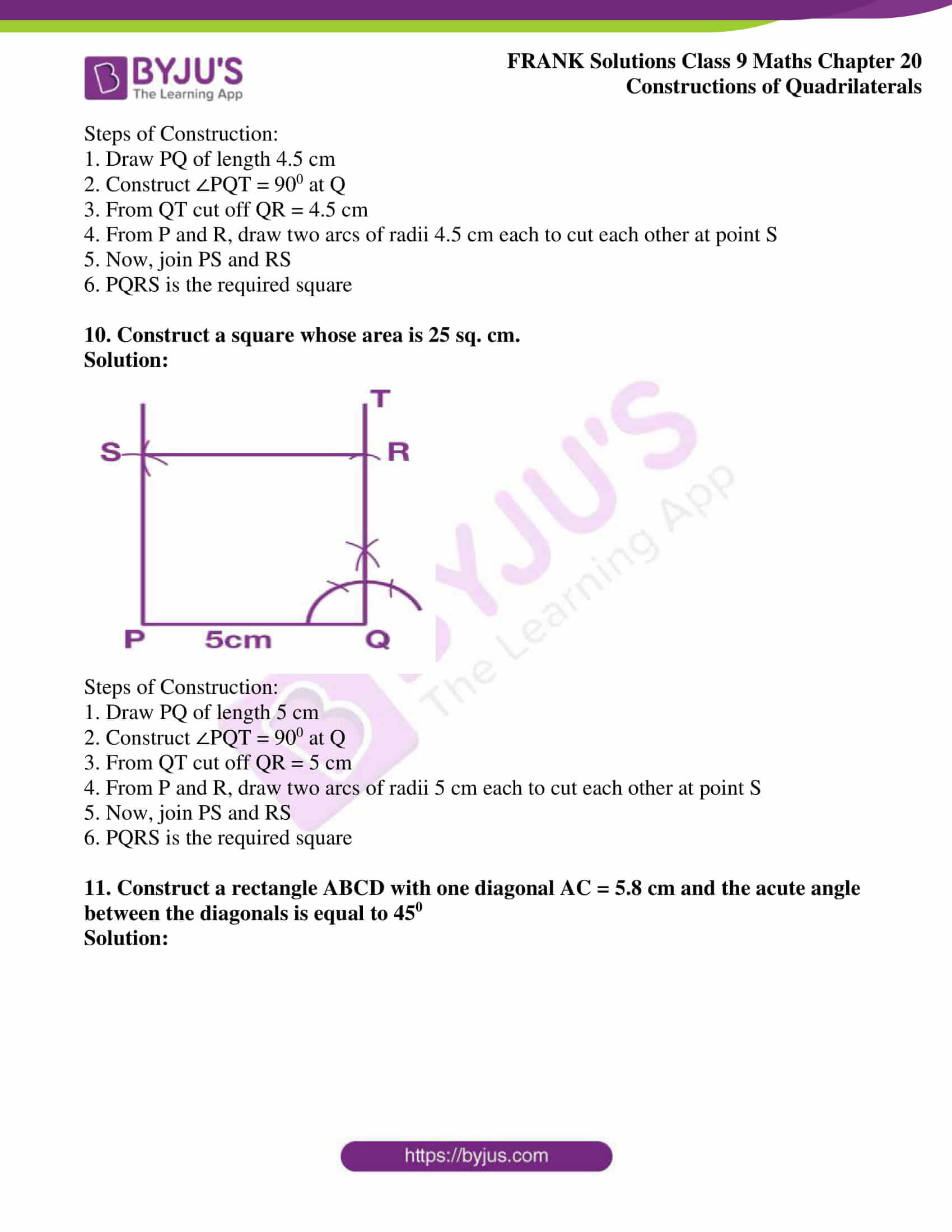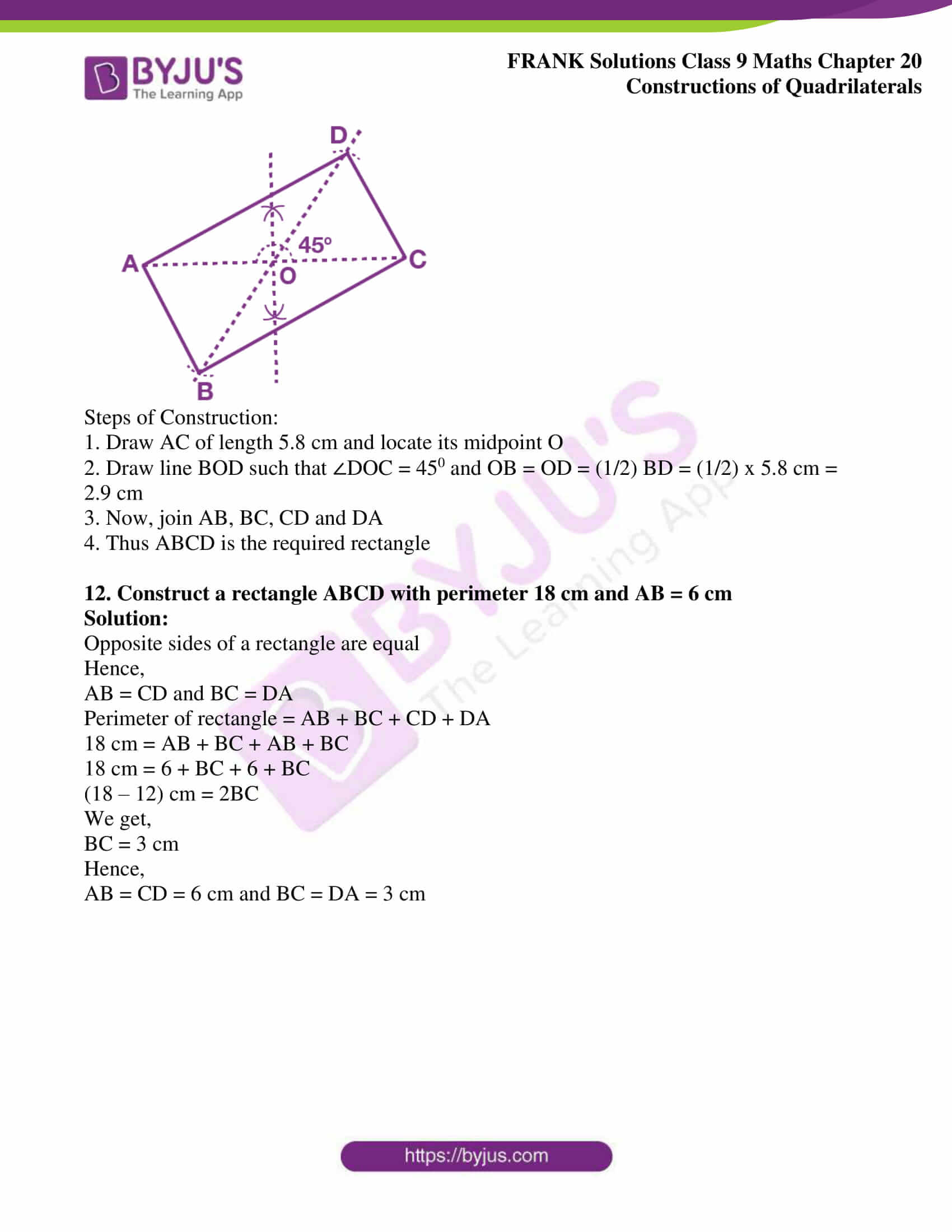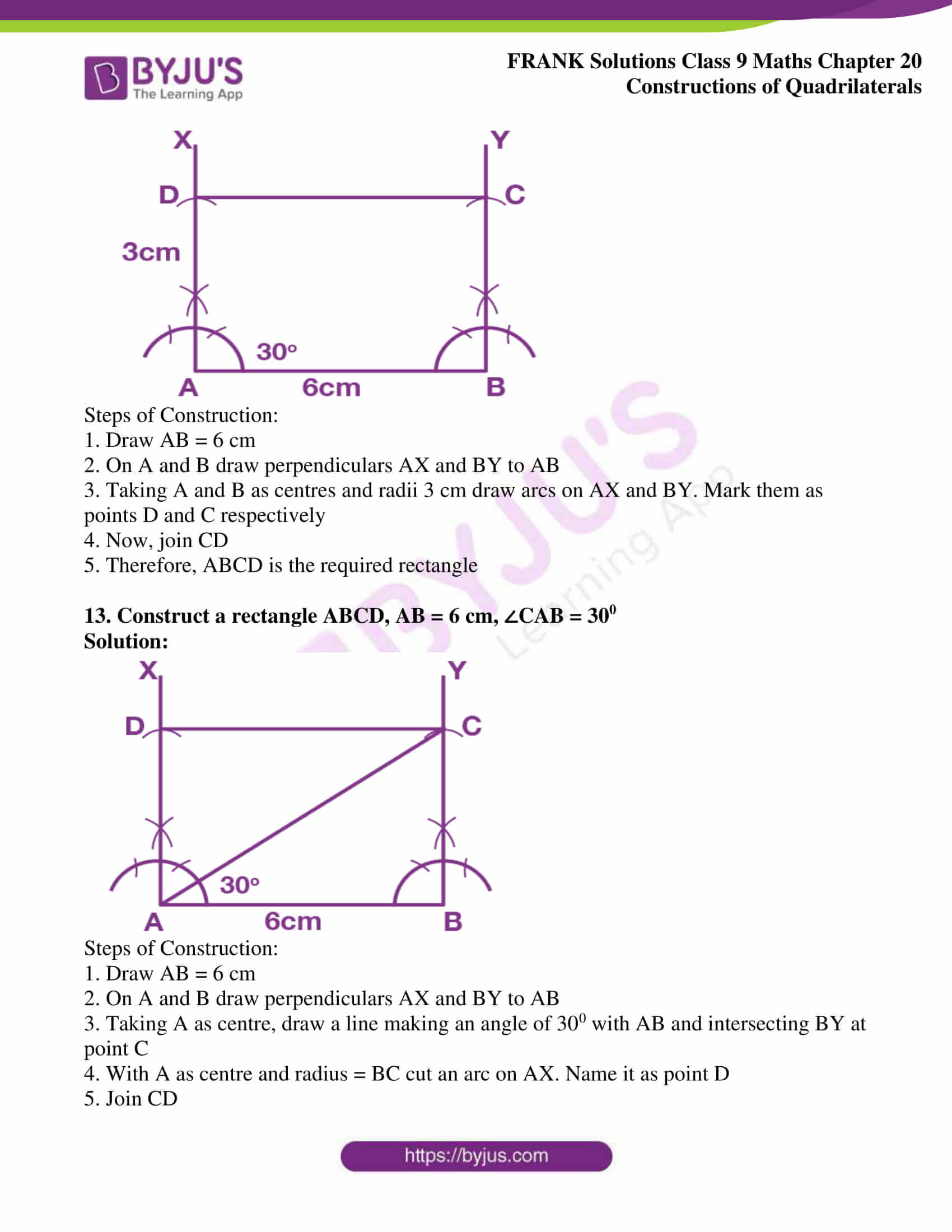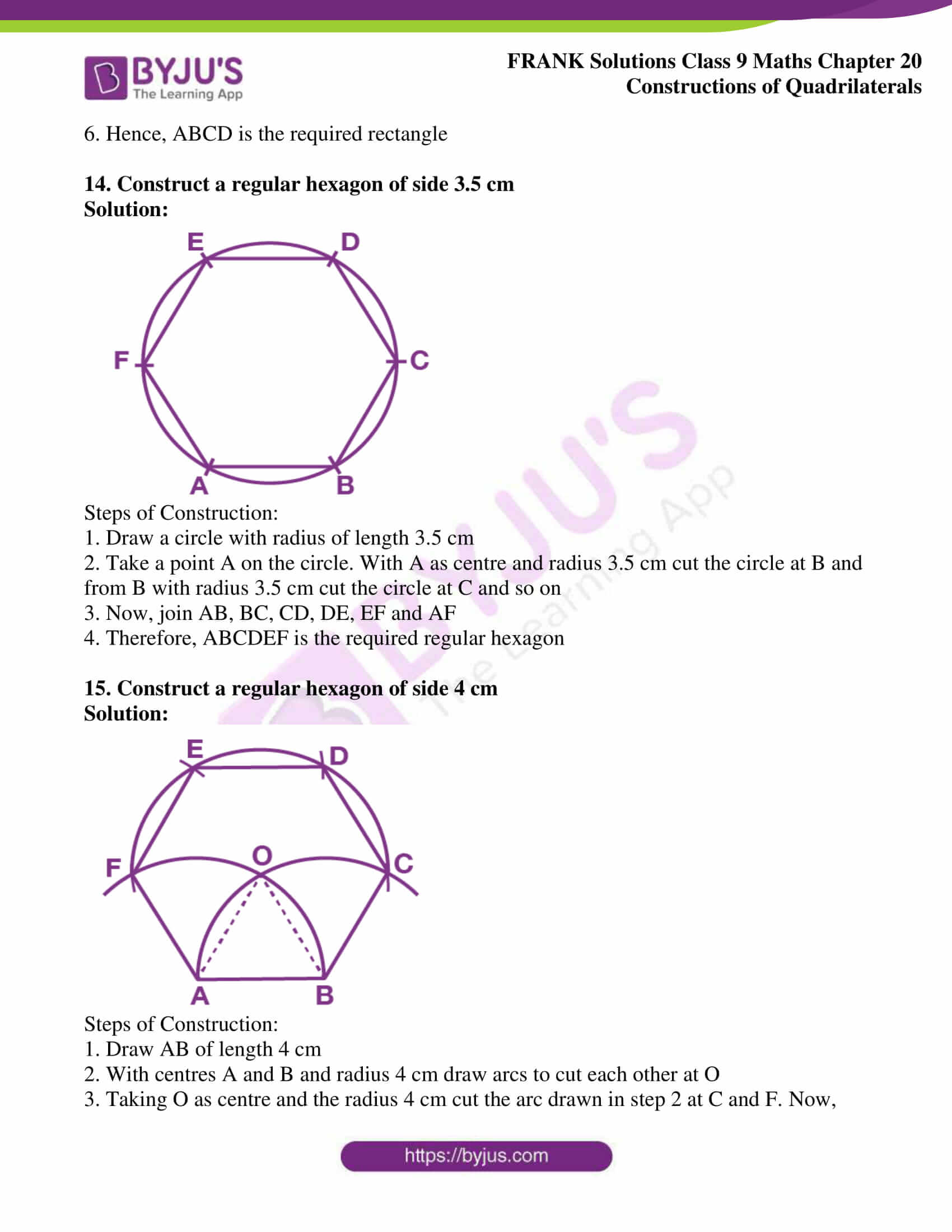## Access Frank Solutions for Class 9 Maths Chapter 20 Constructions of Quadrilaterals

1. Construct a quadrilateral ABCD in which

(i) AB = 4.6 cm, BD = 5 cm, AC = 6 cm, CD = 4.2 cm and ∠A = 900

(ii) AB = 7.2 cm, BC = 5.8 cm, CD = 6.3 cm, AD = 4.3 cm and ∠A = 750

(iii) AB = 4.8 cm, AC = 5.8 cm, AD = 3.6 cm, ∠A = 1050 and ∠B = 600

(iv) AD = AB = 5 cm, BC = 3.8 cm, CD = 3.5 cm and ∠BAD = 450

Solution:

(i) AB = 4.6 cm, BD = 5 cm, AC = 6 cm, CD = 4.2 cm and ∠A = 900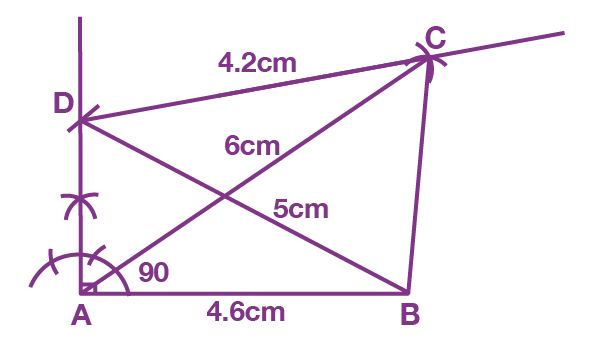Steps of Construction:

1. Draw a line segment AB = 4.6 cm

2. Taking A as centre, draw a ray making an angle of 900 with AB

3. With B as centre and radius equal to 5 cm cut an arc on the ray from A and mark it as point D

4. With D as centre and radius 4.2 cm cut an arc on right side of AD

5. Taking A as centre and radius 6 cm cut an arc which meets the arc from D at point C

6. Now, join BC

7. ABCD is the required quadrilateral

(ii) AB = 7.2 cm, BC = 5.8 cm, CD = 6.3 cm, AD = 4.3 cm and ∠A = 750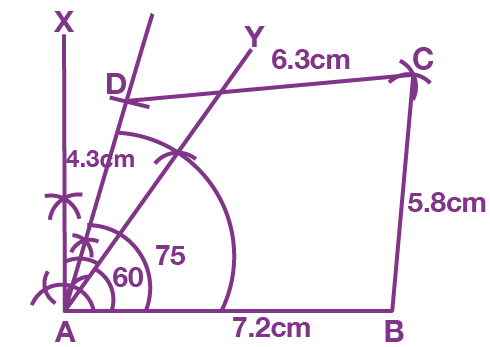Steps of Construction:

1. Draw a line segment AB = 7.2 cm

2. With A as centre draw rays X and Y to make angles 900 and 600 with AB. Then bisect the angle between them to make an angle of 750 with AB

3. With A as centre and radius 4.3 cm cut an arc on line segment making 750 angle with AB and mark it as point D

4. With D and B as centres and radii of 6.3 and 5.8 cm respectively, draw arcs cutting each other at point C

5. Now, join DC and BC

6. ABCD is the required quadrilateral

(iii) AB = 4.8 cm, AC = 5.8 cm, AD = 3.6 cm, ∠A = 1050 and ∠B = 600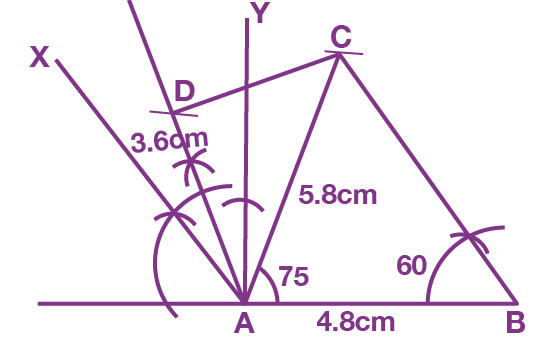Steps of Construction:

1. Draw a line segment AB = 4.8 cm

2. With A as centre draw rays X and Y to make angles 600 and 900 with AB produced. Then bisect the angle between them to make an angle of 1050 with AB

3. With A as centre and radius 3.6 cm cut an arc on line segment making 1050 angle with AB and name it as point D

4. With B as centre draw a ray making an angle of 600 with AB

5. With A as centre and radius 5.8 cm cut an arc on the ray from B and mark the point as C

6. Now, join BC and DC

7. ABCD is the required quadrilateral

(iv) AD = AB = 5 cm, BC = 3.8 cm, CD = 3.5 cm and ∠BAD = 450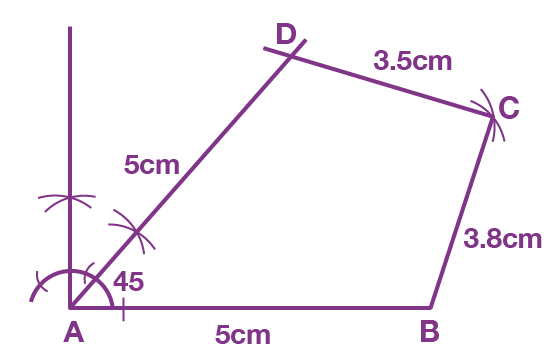Steps of Construction:

1. Draw a line segment AB = 5 cm

2. With A as centre draw an angle of 900 and bisect it to form ∠BAD = 450

3. With A as centre and radius 5 cm cut an arc on the ray making an angle of 450 with AB and name it as point D

4. With D and B as centre and radii as 3.5 cm and 3.8 cm respectively, draw arcs intersecting each other at point C

5. Now, join DC and BC

6. ABCD is the required quadrilateral

2. Construct a trapezium ABCD in which

(i) AB = 3.5 cm, BC = 6 cm, CD = 3.5 cm, AD = 4.4 cm and AD || BC

(ii) AB = 4.6 cm, BC = 6.4 cm, CD = 5.6 cm, ∠B = 600 and AD || BC

Solution:

(i) AB = 3.5 cm, BC = 6 cm, CD = 3.5 cm, AD = 4.4 cm and AD || BC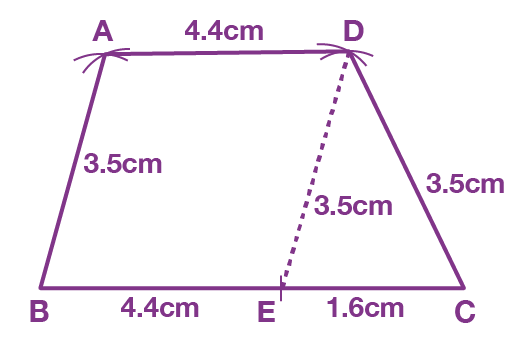Steps of Construction:

1. Draw BC of length 6 cm

2. From BC, cut BE = 4.4 cm

3. Draw a triangle DEC, such that DE = AB = 3.5 cm and CD = 3.5 cm

4. With B and D as centres and radii 3.5 cm and 4.4 cm, respectively, draw arcs cutting each other at point A

5. Now, join AB and AD

6. Thus, ABCD is the required trapezium

(ii) AB = 4.6 cm, BC = 6.4 cm, CD = 5.6 cm, ∠B = 600 and AD || BC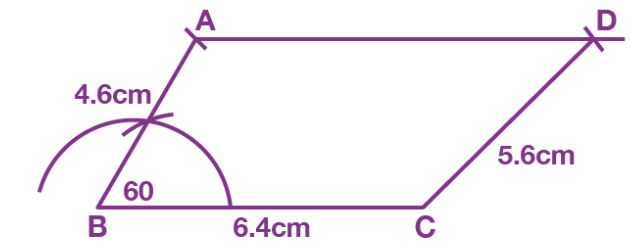Steps of Construction:

1. Draw BC of length 6.4 cm

2. With B as centre, draw an angle of 600 and cut an arc with radius 4.6 cm. Name this point as A

3. From point A, draw a line segment parallel to BC

4. With C as centre and radius 5.6 cm cut an arc on the line segment parallel to BC. Name this point as D

5. Join CD

6. Thus, ABCD is the required trapezium

3. Construct a parallelogram ABCD in which AB = 4.5 cm, ∠A = 1050 and the distance between AB and CD is 3.2 cm

Solution: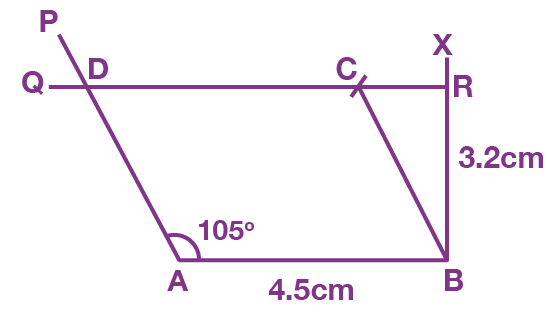Steps of Construction:

1. Draw a line segment AB = 4.5 cm

2. At B, draw BX perpendicular to AB

3. From B, cut BR = 3.2 cm on BX, which is the distance between AB and CD

4. Through R, draw a line perpendicular to BX to get QR parallel to AB

5. Taking A as centre, draw a ray AP making an angle of 1050 with AB and meeting QR at point D

6. Taking B as centre, draw an arc with radius = AD on QR and name it as point C

7. Now, join BC

8. ABCD is the required parallelogram

4. Construct a rhombus whose perimeter is 16 cm and BD = 6.2 cm

Solution: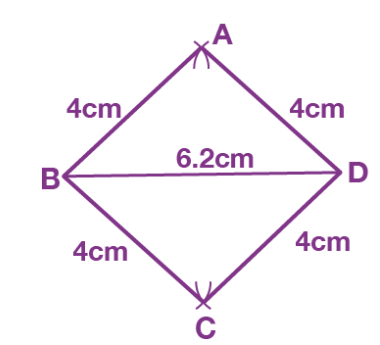The length of all the sides of the rhombus is equal

Therefore,

Perimeter = side x 4

Side = perimeter / 4

Side = 16 / 4

We get,

Side = 4 cm

Steps of Construction:

1. Draw a line segment BD = 6.2 cm

2. Taking B as centre and radius 4 cm, draw two arcs one above BD and the other below BD

3. Taking D as centre and radius 4 cm draw two arcs one above BD and the other below BD, intersecting the previous arcs drawn in step 2 at points A and C, respectively

4. Now, join AB, BC, CD and AD

5. ABCD is the required rhombus

5. Construct a rhombus whose diagonals AC = 7.4 cm and BD = 6 cm

Solution: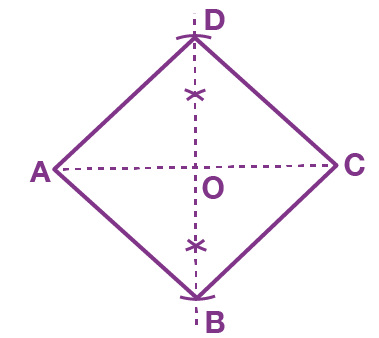The diagonals of a rhombus bisect each other

Steps of Construction:

1. Draw a line segment AC = 7.4 cm

2. Draw perpendicular bisector to AC which cuts AC at point O

3. From this perpendicular, cut OD and OB such that OD = OB = (1/2) BD = (1/ 2) x 6 cm = 3 cm

4. Now, join AB, BC, CD and AD

5. ABCD is the required rhombus

6. Construct a rhombus whose side AB = 5 cm and diagonal AC = 6 cm. Measure DB and AD.

Solution: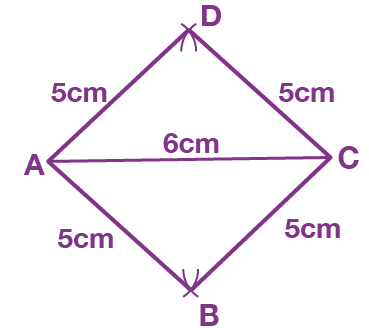In rhombus, all sides are equal

Steps of Construction:

1. Draw AC of length 6 cm

2. With A as centre and radius 5 cm, draw two arcs one above AC and the other below AC

3. Taking C as centre and radius 5 cm draw two arcs one above AC and the other below AC intersecting the previous arcs of Step 2 at points B and D respectively

4. Now, join AB, BC, CD and AD

5. ABCD is the required rhombus

6. On measuring we get, AD = 5 cm and DB = 8 cm

7. Construct a square with each side 4.3 cm

Solution: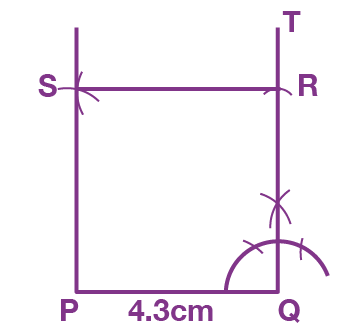Sides of square are equal

Steps of Construction:

1. Draw PQ of length 4.3 cm

2. Construct ∠PQT = 900 at Q

3. From QT cut off QR = 4.3 cm

4. From P and R, draw two arcs of radii 4.3 cm each to cut each other at point S

5. Now, join PS and RS

6. PQRS is the required square

8. Construct a square ABCD with AC = 6.5 cm

Solution: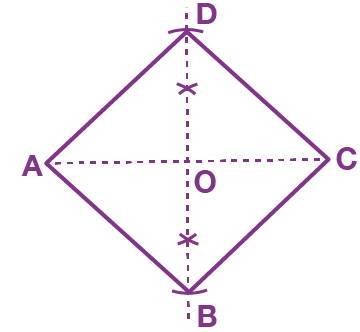The diagonals of a square are equal and bisect each other

Steps of Construction:

1. Draw AC of length 6.5 cm

2. Draw perpendicular bisector to AC which cuts AC at point O

3. From this perpendicular cut OD and OB such that OD = OB = (1/2) BD = (1/2) x 6.5 = 3.25 cm

4. Now, join AB, BC, CD and AD

5. ABCD is the required square

9. Construct a square with perimeter = 18 cm

Solution: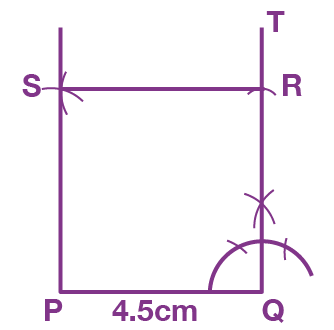Sides of square are equal

Perimeter = 4 x side

Side = perimeter / 4

Side = 18 / 4

We get,

Side = 4.5 cm

Steps of Construction:

1. Draw PQ of length 4.5 cm

2. Construct ∠PQT = 900 at Q

3. From QT cut off QR = 4.5 cm

4. From P and R, draw two arcs of radii 4.5 cm each to cut each other at point S

5. Now, join PS and RS

6. PQRS is the required square

10. Construct a square whose area is 25 sq. cm.

Solution: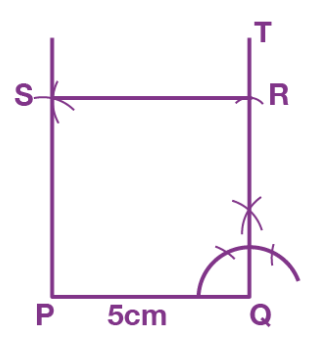Steps of Construction:

1. Draw PQ of length 5 cm

2. Construct ∠PQT = 900 at Q

3. From QT cut off QR = 5 cm

4. From P and R, draw two arcs of radii 5 cm each to cut each other at point S

5. Now, join PS and RS

6. PQRS is the required square

11. Construct a rectangle ABCD with one diagonal AC = 5.8 cm and the acute angle between the diagonals is equal to 450

Solution: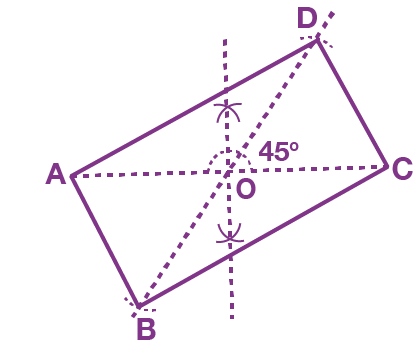Steps of Construction:

1. Draw AC of length 5.8 cm and locate its midpoint O

2. Draw line BOD such that ∠DOC = 450 and OB = OD = (1/2) BD = (1/2) x 5.8 cm = 2.9 cm

3. Now, join AB, BC, CD and DA

4. Thus ABCD is the required rectangle

12. Construct a rectangle ABCD with perimeter 18 cm and AB = 6 cm

Solution:

Opposite sides of a rectangle are equal

Hence,

AB = CD and BC = DA

Perimeter of rectangle = AB + BC + CD + DA

18 cm = AB + BC + AB + BC

18 cm = 6 + BC + 6 + BC

(18 – 12) cm = 2BC

We get,

BC = 3 cm

Hence,

AB = CD = 6 cm and BC = DA = 3 cm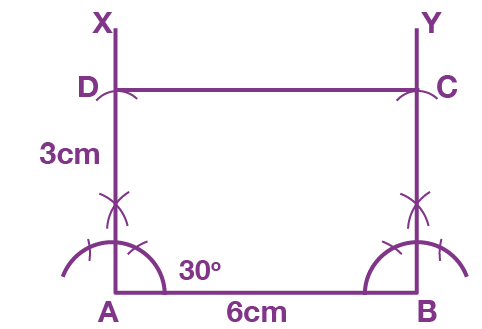Steps of Construction:

1. Draw AB = 6 cm

2. On A and B draw perpendiculars AX and BY to AB

3. Taking A and B as centres and radii 3 cm draw arcs on AX and BY. Mark them as points D and C, respectively

4. Now, join CD

5. Therefore, ABCD is the required rectangle

13. Construct a rectangle ABCD, AB = 6 cm, ∠CAB = 300

Solution: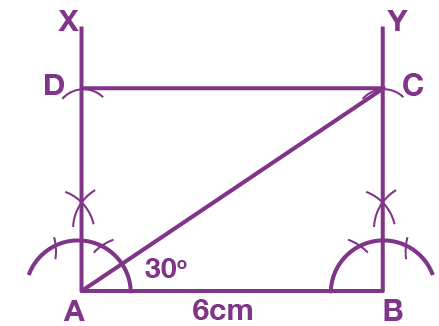Steps of Construction:

1. Draw AB = 6 cm

2. On A and B, draw perpendiculars AX and BY to AB

3. Taking A as centre, draw a line making an angle of 300 with AB and intersecting BY at point C

4. With A as centre and radius = BC, cut an arc on AX. Name it as point D

5. Join CD

6. Hence, ABCD is the required rectangle

14. Construct a regular hexagon of side 3.5 cm

Solution: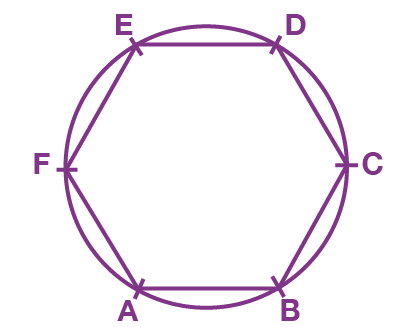Steps of Construction:

1. Draw a circle with radius of length 3.5 cm

2. Take a point A on the circle. With A as centre and radius 3.5 cm cut the circle at B and from B with radius 3.5 cm cut the circle at C and so on

3. Now, join AB, BC, CD, DE, EF and AF

4. Therefore, ABCDEF is the required regular hexagon

15. Construct a regular hexagon of side 4 cm

Solution: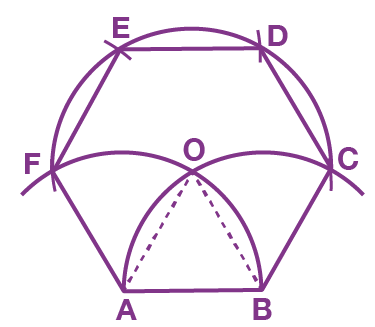Steps of Construction:

1. Draw AB of length 4 cm

2. With centres A and B and radius 4 cm draw arcs to cut each other at O

3. Taking O as centre and the radius 4 cm cut the arc drawn in step 2 at C and F. Now, join AF and BC

4. With centres C and F and radius 4 cm cut the arcs drawn in step 3 at D and E. Then join CD, DE and EF

5. Thus, ABCDEF is the required regular hexagon